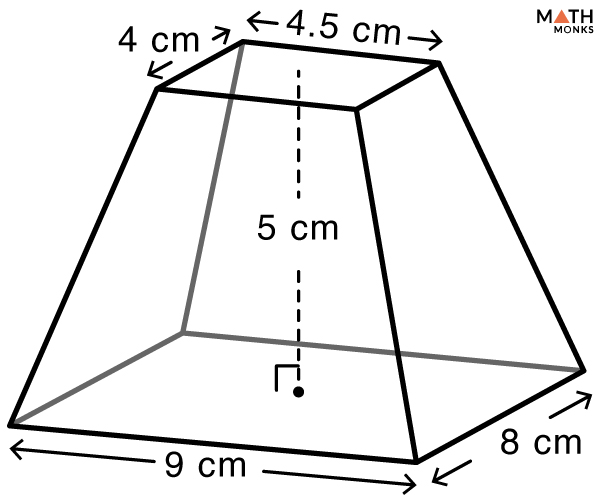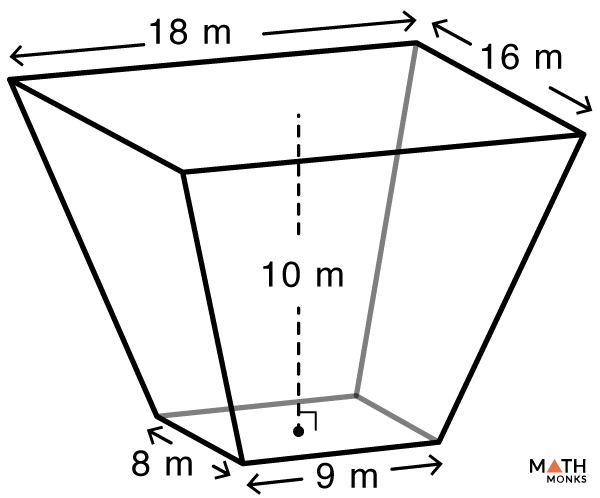# Truncated Pyramid (Frustum of a Pyramid )

A truncated pyramid is a pyramid that is chopped off from the tip with a plane parallel to its base.

## Parts

1. 2 Bases – Polygon-shaped, one at the top, and one at the bottom. They are always of the same polygonal shape. For example, a truncated triangular pyramid has 2 triangular bases.
2. Side faces – The lateral faces bounding the bases. They are always trapezoidal.
3. Height – The distance between the centers of the 2 bases. It is represented by ‘h’.
4. Slant height – The shortest distance between the outer edges of the bases. The slant height of a regular truncated pyramid is also known as its apothem. It is represented by ‘l’.

## Formulas

### Volume

The formula is:

Calculate the volume of a truncated pyramid with lower base of 5 cm, and upper base of 3 cm, and a height of 6 cm.

Solution:

As we know,
Volume (V) = ${\dfrac{1}{3}h\left( a^{2}+b^{2}+ab\right)}$, here a = 5 cm, b = 3 cm, h = 6 cm
∴ V = ${\dfrac{1}{3}\times 6\times \left( 5^{2}+3^{2}+5\times3\right)}$
= 98 cm3Find the volume of a given truncated square pyramid.

Solution:

As we know,
Volume (V) = ${\dfrac{1}{3}\times h\times \left( a^{2}+b^{2}+ab\right)}$, here a = 5 cm, b = 3 cm, h = 4 cm
∴ V = ${\dfrac{1}{3}\times 4\times \left( 5^{2}+3^{2}+5\times 3\right)}$
= 65.33 cm3

Finding the volume of a truncated rectangular pyramid when lower base length, lower base width, upper base length, upper base width, and height are knownFind the volume of a truncated rectangular pyramid given in the figure.

Solution:

As we know,
Volume (V) = ${\dfrac{Ab+aB+2\left( ab+AB\right) }{6}\times h}$, here A = 9 cm, B = 8 cm, a = 4.5 cm, b = 4 cm, h = 5 cm
V = ${\dfrac{9\times 4+4.5\times 8+2\left( 4.5\times 4+9\times 8\right) }{6}\times 5}$
= 210 cm3Find the volume of a truncated rectangular pyramid pond given in the figure.

Solution:

As we know,
Volume (V) = ${\dfrac{Ab+aB+2\left( ab+AB\right) }{6}\times h}$, here A = 18 m, B = 16 m, a = 9 m, b = 8 m, h = 10 m
V = ${\dfrac{18\times 8+9\times 16+2\left( 9\times 8+18\times 16\right) }{6}\times 10}$
= 1680 m3

### Surface Area

The formula is:

Surface Area (SA) = ${\dfrac{P_{1}+P_{2}}{2}\times l+B_{1}+B_{2}}$, here P1 & P2 = base perimeters, B1 & B2 = base areas, l = slant height, ${\dfrac{P_{1}+P_{2}}{2}\times l}$ = Lateral Surface Area (LSA)

Lateral Surface Area (LSA) = area of the lateral faces only.

${\therefore TSA=LSA+B_{1}+B_{2}}$

Let us solve an example to understand the concept better.

Calculate the lateral and total surface area of a truncated pyramid with base perimeters are 28 cm and 24 cm, an apothem of 6 cm, and base areas of 49 cm2 and 36 cm2.

Solution:

As we know,
Lateral Surface Area (LSA) = ${\dfrac{P_{1}+P_{2}}{2}\times l}$, here P1  = 24 cm, P2 = 28 cm, l = 6 cm
${\therefore LSA=\dfrac{24+28}{2}\times 6}$
${=156cm^{2}}$
Total Surface Area (TSA) = ${LSA+B_{1}+B_{2}}$, here LSA = 156 cm2, B1 = 36 cm2, B2 = 49 cm2
${\therefore TSA=156+36+49}$
${=241cm^{2}}$